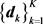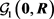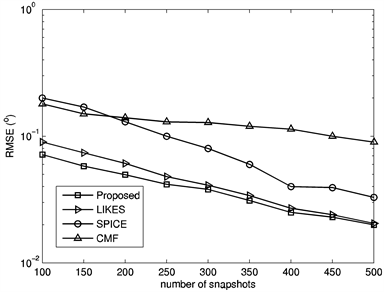# 一种基于压缩感知的无需超参数的测向算法A Hyperparameter-Free Direction-Finding Algorithm in the Compressive Sensing Framework

• 全文下载: PDF(621KB)    PP.1-7   DOI: 10.12677/HJWC.2019.91001
• 下载量: 394  浏览量: 520

The fully automatic sparsity-parameter estimation algorithms do not require the user to make any hard decision (possibly via trial-and-error) on the values of the hyperparameters, making them more pragmatic in practice. This paper provides a unified interpretation of the existing approaches including covariance matrix fitting (CMF), sparse iterative covariance based estimation (SPICE) and likelihood-based estimation of sparse parameters (LIKES). The point of view taken is that they are all covariance-fitting-based algorithms under different statistical distances. Following this, we present a new covariance-fitting scheme trying to minimize one of the two asymmetrical Itakura-Saito distances. Simulations show that the proposed method appears to be preferable as it outperforms the aforementioned algorithms in general.

1. 引言

2. 数学模型

$x\left(t\right)={\sum }_{m=1}^{M}{a}_{m}{s}_{m}\left(t\right)+n\left(t\right)$ (1)

${\left\{{\theta }_{k}\right\}}_{k=1}^{K}$ 为可以覆盖所有可能入射区域的栅格，并覆盖了(至少是近似覆盖了)上述M个信号的入射角度，相应的导向矢量为，其中， $K\gg N$ 。于是，式(1)可以重新表述为：

$x\left(t\right)={\sum }_{k=1}^{K}{d}_{k}{\stackrel{¯}{s}}_{k}\left(t\right)+n\left(t\right)$ (2)

$R=E\left\{x\left(t\right){x}^{\text{H}}\left(t\right)\right\}={\sum }_{k=1}^{K}{\sigma }_{k}{d}_{k}{d}_{k}^{\text{H}}+ϵI$ (3)

$R={\sum }_{k=1}^{K+N}{\sigma }_{k}{d}_{k}{d}_{k}^{\text{H}}$ (4)

$\stackrel{^}{R}=\frac{1}{L}{\sum }_{l=1}^{L}x\left[l\right]{x}^{\text{H}}\left[l\right]$ (5)

2.1. 协方差矩阵拟合算法(CMF)

$\begin{array}{l}\underset{{\left\{{\sigma }_{k}\right\}}_{k=1}^{K},ϵ}{\mathrm{min}}\left\{{d}_{\text{SE}}\left(\stackrel{^}{R},R\right)={‖\stackrel{^}{R}-\underset{k=1}{\overset{K}{\sum }}{\sigma }_{k}{d}_{k}{d}_{k}^{\text{H}}-ϵI‖}^{2}\right\}\\ \text{s}.\text{t}.\text{\hspace{0.17em}}\text{\hspace{0.17em}}{\left\{{\sigma }_{k}\right\}}_{k=1}^{K}\ge 0,\text{\hspace{0.17em}}\text{\hspace{0.17em}}{\sum }_{k=1}^{K}{\sigma }_{k}\le \lambda ,\text{\hspace{0.17em}}\text{\hspace{0.17em}}\lambda =-N{\gamma }_{N}+{\sum }_{n=1}^{N}{\gamma }_{n},\text{\hspace{0.17em}}\text{\hspace{0.17em}}ϵ\ge 0\end{array}$ (6)

$\begin{array}{l}\underset{{\left\{{\sigma }_{k}\right\}}_{k=1}^{K+N}}{\mathrm{min}}\left\{{d}_{\text{SE}}\left(\stackrel{^}{R},R\right)={‖\stackrel{^}{R}-\underset{k=1}{\overset{K+N}{\sum }}{\sigma }_{k}{d}_{k}{d}_{k}^{\text{H}}‖}^{2}\right\}\\ \text{s}.\text{t}.\text{\hspace{0.17em}}\text{\hspace{0.17em}}{\left\{{\sigma }_{k}\right\}}_{k=1}^{K+N}\ge 0\end{array}$ (7)

2.2. 基于稀疏迭代协方差的估计(SPICE)

SPICE算法  实际上是将两种高斯分布之间的Jeffreys距离(亦称为对称Kullback-Leibler距离)  最小化：

$\begin{array}{l}\underset{{\left\{{\sigma }_{k}\right\}}_{k=1}^{K+N}}{\mathrm{min}}\left\{{‖{R}^{-1/2}\left(\stackrel{^}{R}-R\right){\stackrel{^}{R}}^{-1/2}‖}^{2}=\text{tr}\left({R}^{-1}\stackrel{^}{R}\right)+\text{tr}\left({\stackrel{^}{R}}^{-1}R\right)-2N=2{d}_{KL}\left(\stackrel{^}{R},R\right)\right\}\\ \text{s}.\text{t}.\text{\hspace{0.17em}}\text{\hspace{0.17em}}\text{\hspace{0.17em}}R={\sum }_{k=1}^{K+N}{\sigma }_{k}{d}_{k}{d}_{k}^{\text{H}},\text{\hspace{0.17em}}\text{\hspace{0.17em}}\text{\hspace{0.17em}}{\left\{{\sigma }_{k}\right\}}_{k=1}^{K+N}\ge 0\end{array}$ (8)

2.3. 基于似然的稀疏参数估计(LIKES)

LIKES算法  使用的是高斯最大似然(GML)标准：

$\begin{array}{l}\underset{{\left\{{\sigma }_{k}\right\}}_{k=1}^{K+N}}{\mathrm{min}}\left\{{X}^{\text{H}}{R}^{-1}X+\text{ln}|R|\right\}\\ \text{s}.\text{t}\text{.}\text{\hspace{0.17em}}\text{\hspace{0.17em}}\text{\hspace{0.17em}}R={\sum }_{k=1}^{K+N}{\sigma }_{k}{d}_{k}{d}_{k}^{\text{H}},\text{\hspace{0.17em}}\text{\hspace{0.17em}}\text{\hspace{0.17em}}{\left\{{\sigma }_{k}\right\}}_{k=1}^{K+N}\ge 0\end{array}$ (9)

$\begin{array}{l}\underset{{\left\{{\sigma }_{k}\right\}}_{k=1}^{K+N}}{\mathrm{min}}\left\{{d}_{\text{IS}}\left(\stackrel{^}{R},R\right)=\text{tr}\left(\stackrel{^}{R}{R}^{-1}-I\right)-\text{ln}|\stackrel{^}{R}{R}^{-1}|\right\}\\ \text{s}.\text{t}\text{.}\text{\hspace{0.17em}}\text{\hspace{0.17em}}\text{\hspace{0.17em}}R={\sum }_{k=1}^{K+N}{\sigma }_{k}{d}_{k}{d}_{k}^{\text{H}},\text{\hspace{0.17em}}\text{\hspace{0.17em}}\text{\hspace{0.17em}}{\left\{{\sigma }_{k}\right\}}_{k=1}^{K+N}\ge 0\end{array}$ (10)

3. 本文提出的协方差矩阵拟合方案

$\begin{array}{l}\underset{{\left\{{\sigma }_{k}\right\}}_{k=1}^{K+N}}{\mathrm{min}}\left\{{d}_{\text{IS}}\left(R,\stackrel{^}{R}\right)=\text{tr}\left(R{\stackrel{^}{R}}^{-1}-I\right)-\text{ln}|R{\stackrel{^}{R}}^{-1}|\right\}\\ \text{s}.\text{t}\text{.}\text{\hspace{0.17em}}\text{\hspace{0.17em}}\text{\hspace{0.17em}}R={\sum }_{k=1}^{K+N}{\sigma }_{k}{d}_{k}{d}_{k}^{\text{H}},\text{\hspace{0.17em}}\text{\hspace{0.17em}}\text{\hspace{0.17em}}{\left\{{\sigma }_{k}\right\}}_{k=1}^{K+N}\ge 0\end{array}$ (11)

${d}_{\text{IS}}\left(R,\stackrel{^}{R}\right)=\text{tr}\left(R{\stackrel{^}{R}}^{-1}\right)-\text{ln}|R|+\text{const}={\sum }_{k=1}^{K+N}{\sigma }_{k}{d}_{k}^{\text{H}}{\stackrel{^}{R}}^{-1}{d}_{k}-\text{ln}|{\sum }_{k=1}^{K+N}{\sigma }_{k}{d}_{k}{d}_{k}^{\text{H}}|+\text{const}$ (12)

$\stackrel{¯}{R}=\stackrel{^}{\alpha }I+\stackrel{^}{\beta }\stackrel{^}{R}$ (13)

$\stackrel{^}{\rho }=\frac{1}{{L}^{2}}\underset{l=1}{\overset{L}{\sum }}{‖x\left[l\right]‖}^{4}-\frac{1}{L}{‖\stackrel{^}{R}‖}^{2}$

$\stackrel{^}{\nu }=\frac{\text{tr}\left(\stackrel{^}{R}\right)}{N}$

$\stackrel{^}{\beta }=1-\frac{\stackrel{^}{\alpha }}{\stackrel{^}{\nu }}$ (14)

$\begin{array}{l}\underset{{\left\{{\sigma }_{k}\right\}}_{k=1}^{K+N}}{\mathrm{min}}\left\{\underset{k=1}{\overset{K+N}{\sum }}{\sigma }_{k}{d}_{k}^{\text{H}}{\stackrel{^}{R}}^{-1}{d}_{k}-\text{ln}|\underset{k=1}{\overset{K+N}{\sum }}{\sigma }_{k}{d}_{k}{d}_{k}^{\text{H}}|\right\}\\ \text{s}.\text{t}.\text{\hspace{0.17em}}\text{\hspace{0.17em}}\text{\hspace{0.17em}}{\left\{{\sigma }_{k}\right\}}_{k=1}^{K+N}\ge 0\end{array}$ (15)

$c={\left[{d}_{1}^{\text{H}}{\stackrel{^}{R}}^{-1}{d}_{1},{d}_{2}^{\text{H}}{\stackrel{^}{R}}^{-1}{d}_{2},\cdots ,{d}_{K+N}^{\text{H}}{\stackrel{^}{R}}^{-1}{d}_{K+N}\right]}^{\text{T}}$ (16)

$p={\left[{\sigma }_{1},{\sigma }_{2},\cdots ,{\sigma }_{K+N}\right]}^{\text{T}}$ (17)

$D=\left[{d}_{1},{d}_{2},\cdots ,{d}_{K+N}\right]$ (18)

cvx_begin quiet

cvx_precision best

cvx_expert true

variable p(K+N,1)

minimize (c'*p-log_det(D*diag(p)*D'))

subject to

p >= zeros(K+N,1);

cvx_end

4. 计算机仿真

$\text{RMSE}=\sqrt{\frac{{\sum }_{n=1}^{300}\left[{\left({\stackrel{^}{\theta }}_{1,n}-{\theta }_{1}\right)}^{2}+{\left({\stackrel{^}{\theta }}_{2,n}-{\theta }_{2}\right)}^{2}+{\left({\stackrel{^}{\theta }}_{3,n}-{\theta }_{3}\right)}^{2}\right]}{900}}$ (19)Figure 1. RMSE versus varied SNRFigure 2. RMSE versus varied numbers of samples

5. 结论

  ITU (2011) Spectrum Monitoring Handbook. ITU, Geneva.  Capon, J. (1969) High-Resolution Frequency-Number Spectrum Analysis. Proceedings of the IEEE, 57, 1408-1418. https://doi.org/10.1109/PROC.1969.7278  Schmidt, R.O. (1986) Multiple Emitter Location and Signal Parameter Estimation. IEEE Transactions on Antennas and Propagation, 34, 276-280. https://doi.org/10.1109/TAP.1986.1143830  Malioutov, D., ?etin, M. and Willsky, A. (2005) A Sparse Signal Reconstruction Perspective for Source Localization with Sensor Arrays. IEEE Transactions on Signal Processing, 53, 3010-3022. https://doi.org/10.1109/TSP.2005.850882  Yardibi, T., Li, J., Stoica, P., et al. (2008) Sparsity Constrained Deconvolution Approaches for Acoustic Source Mapping. Journal of Acoustical Society of America, 123, 2631-2642. https://doi.org/10.1121/1.2896754  Stoica, P., Babu, P. and Li, J. (2011) SPICE: A Sparse Covariance-Based Estimation Method for Array Signal Processing. IEEE Transactions on Signal Processing, 59, 629-638. https://doi.org/10.1109/TSP.2010.2090525  Stoica, P. and Babu, P. (2012) SPICE and LIKES: Two Hyperparameter-Free Methods for Sparse-Parameter Estimation. Signal Processing, 92, 1580-1590. https://doi.org/10.1016/j.sigpro.2011.11.010  Tibshirani, R. (1996) Regression Shrinkage and Selection via the Lasso. Journal of Royal Statistical Society: Series B (Statistical Methodology), 58, 267-288. https://doi.org/10.1111/j.2517-6161.1996.tb02080.x  Gorodnitsky, I.F. and Rao, B.D. (1997) Sparse Signal Reconstruction from Limited Data Using FOCUSS: A Re-Weighted Minimum Norm Algorithm. IEEE Transactions on Signal Processing, 45, 600-616. https://doi.org/10.1109/78.558475  Cotter, S.F., Rao, B.D., Engan, K., et al. (2005) Sparse Solutions to Linear Inverse Problems with Multiple Measurement Vectors. IEEE Transactions on Signal Processing, 53, 2477-2488. https://doi.org/10.1109/TSP.2005.849172  Xu, D.Y., Hu, N., Ye, Z.F., et al. (2012) The Estimate for DOAs of Signals Using Sparse Recovery Method. Proceedings of the 37th IEEE International Conference on Acoustics, Speech, and Signal Processing, Kyoto, 2573-2576. https://doi.org/10.1109/ICASSP.2012.6288442  Ottersten, B., Stoica, P. and Roy, R. (1998) Covariance Matching Estima-tion Techniques for Array Signal Processing Applications. Digital Signal Processing, 8, 185-210. https://doi.org/10.1006/dspr.1998.0316  Kullback, S. (1997) Information Theory and Statistics. Dover Edition. Dover, New York.  Bensaid, S. and Slock, D. (2012) Blind Audio Source Separation Exploiting Periodicity and Spectral Envelops. Pro-ceedings of International Workshop on Acoustic Signal Enhancement, Aachen.  Vandenberghe, L., Boyd, S. and Wu, S.P. (1998) Determinant Maximization with Linear Matrix Inequality Constraints. SIAM Journal on Matrix Analysis and Applications, 19, 499-533. https://doi.org/10.1137/S0895479896303430  Landi, L., De Maio, A., De Nicola, S., et al. (2008) Knowledge-Aided Covariance Matrix Estimation: A MAXDET Approach. IET Radar, Sonar & Navigation, 3, 341-356. https://doi.org/10.1109/RADAR.2008.4720823  Li, J., Du, L. and Stoica, P. (2008) Fully Automatic Computation of Di-agonal Loading Levels for Robust Adaptive Beamforming. Proceedings of the 33rd IEEE International Conference on Acoustics, Speech, and Signal Processing, Las Vegas, 2325-2328.  Wu, S.P., Vandenberghe, L. and Boyd, S. (1996) MAXDET: Software for Determinant Maximization Problems. Information Systems Laboratory, Stanford University, Stanford.  Grant, M. and Boyd, S. (2012) CVX: MATLAB Software for Disciplined Convex Programming, Version 2.0 Beta. http://cvxr.com/cvx  Bhattacharyya, A. (1943) On a Measure of Divergence between Two Statistical Populations Defined by Their Probability Distributions. Bulletin of the Calcutta Mathematical Society, 35, 99-109.  Zolotarev, V.M. (1984) Probability Metrics. Theory of Probability and Its Applications, 28, 278-302. https://doi.org/10.1137/1128025